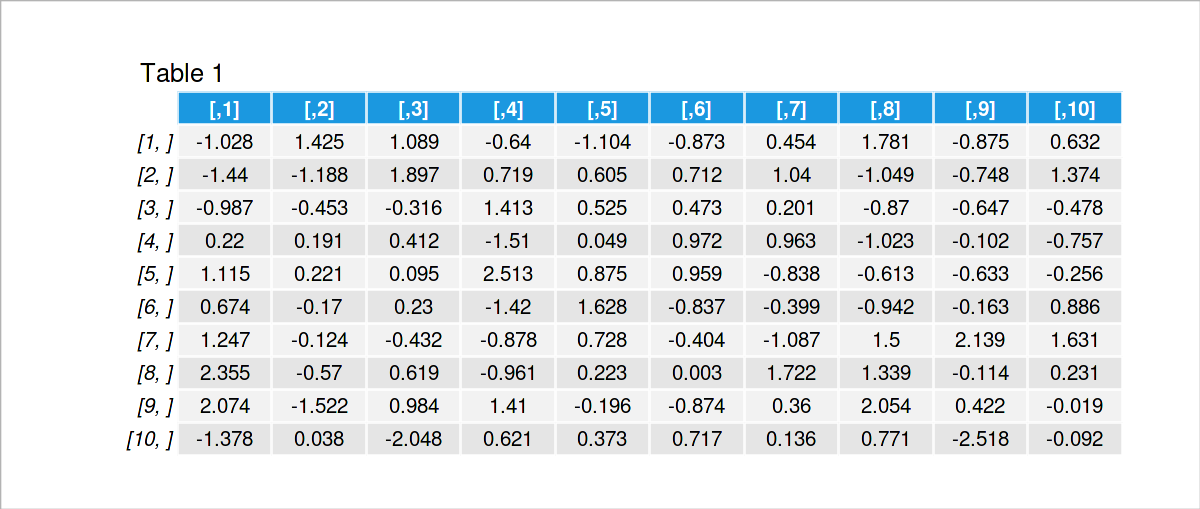# Change Font Size in corrplot in R (3 Examples)

This article demonstrates how to adjust the font size in a corrplot in the R programming language.

So without further additions, let’s get started!

## Example Data, Packages & Default Plot

The first step is to construct some data that we can use in the exemplifying syntax below:

```set.seed(359478)                        # Create example data
data <- matrix(rnorm(100), ncol = 10)
data                                    # Print example data```As you can see based on Table 1, our example data is a matrix having ten rows and ten columns.

To be able to use the functions of the corrplot package, we also need to install and load corrplot:

```install.packages("corrplot")            # Install & load corrplot package
library("corrplot")```

As a next step, we can draw our data in a corrplot correlation matrix with default font sizes:

`corrplot(cor(data), addCoef.col = 1)    # Draw corrplot with default font size`The following examples will show how to change the font sizes of different elements of this corrplot. Keep on reading!

## Example 1: Adjust Font Size of Correlation Coefficients in corrplot

This example shows how to decrease the text size of the correlation coefficients in a corrplot.

For this, we have to specify a smaller value to the number.cex argument. The smaller this value is, the smaller is the font size (or the larger).

Have a look at the following R code and its output:

```corrplot(cor(data), addCoef.col = 1,    # Change font size of correlation coefficients
number.cex = 0.5)```As you can see, we have modified the size of the correlation coefficients in our plot.

## Example 2: Adjust Font Size of Text Labels in corrplot

The following code shows how to change the font size of the text labels at the axes of our corrplot (i.e. the variable names).

To do this, we have to specify a different value for the tl.cex argument:

```corrplot(cor(data), addCoef.col = 1,    # Change font size of text labels
tl.cex = 0.5)```## Example 3: Adjust Font Size of Number-Labels in Color-Legend in corrplot

In Example 3, I’ll show how to change the text size of the legend labels.

In this example, we have to modify the cl.cex argument:

```corrplot(cor(data), addCoef.col = 1,    # Change font size of number-labels in color-legend
cl.cex = 0.5)```## Video, Further Resources & Summary

Do you want to learn more about the adjustment of the font size of different numbers in a corrplot? Then you may want to have a look at the following video which I have published on my YouTube channel. In the video, I demonstrate the R programming codes of this tutorial in a live session.

Please accept YouTube cookies to play this video. By accepting you will be accessing content from YouTube, a service provided by an external third party.If you accept this notice, your choice will be saved and the page will refresh.

In addition, you may have a look at the other tutorials on this website. I have published several related articles already:

Summary: At this point you should have learned how to increase or decrease the font size in a corrplot in R. Don’t hesitate to let me know in the comments section, if you have further questions.

Subscribe to the Statistics Globe Newsletter

•